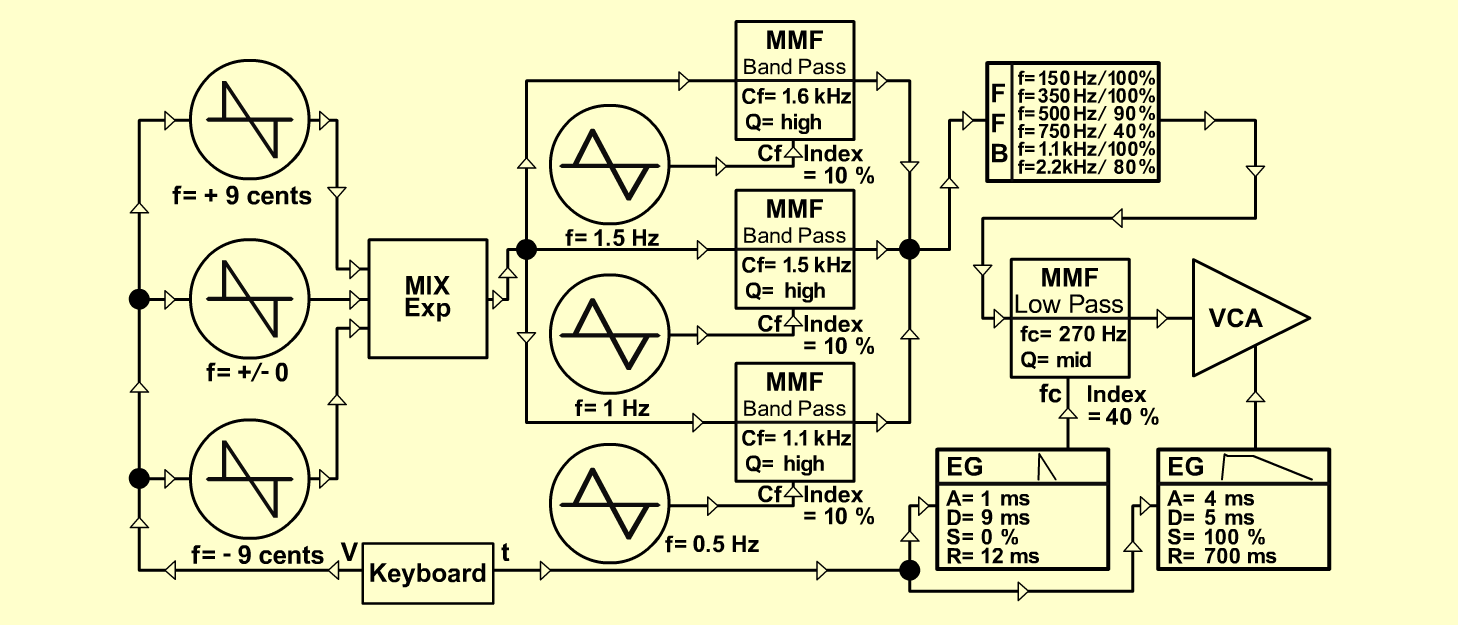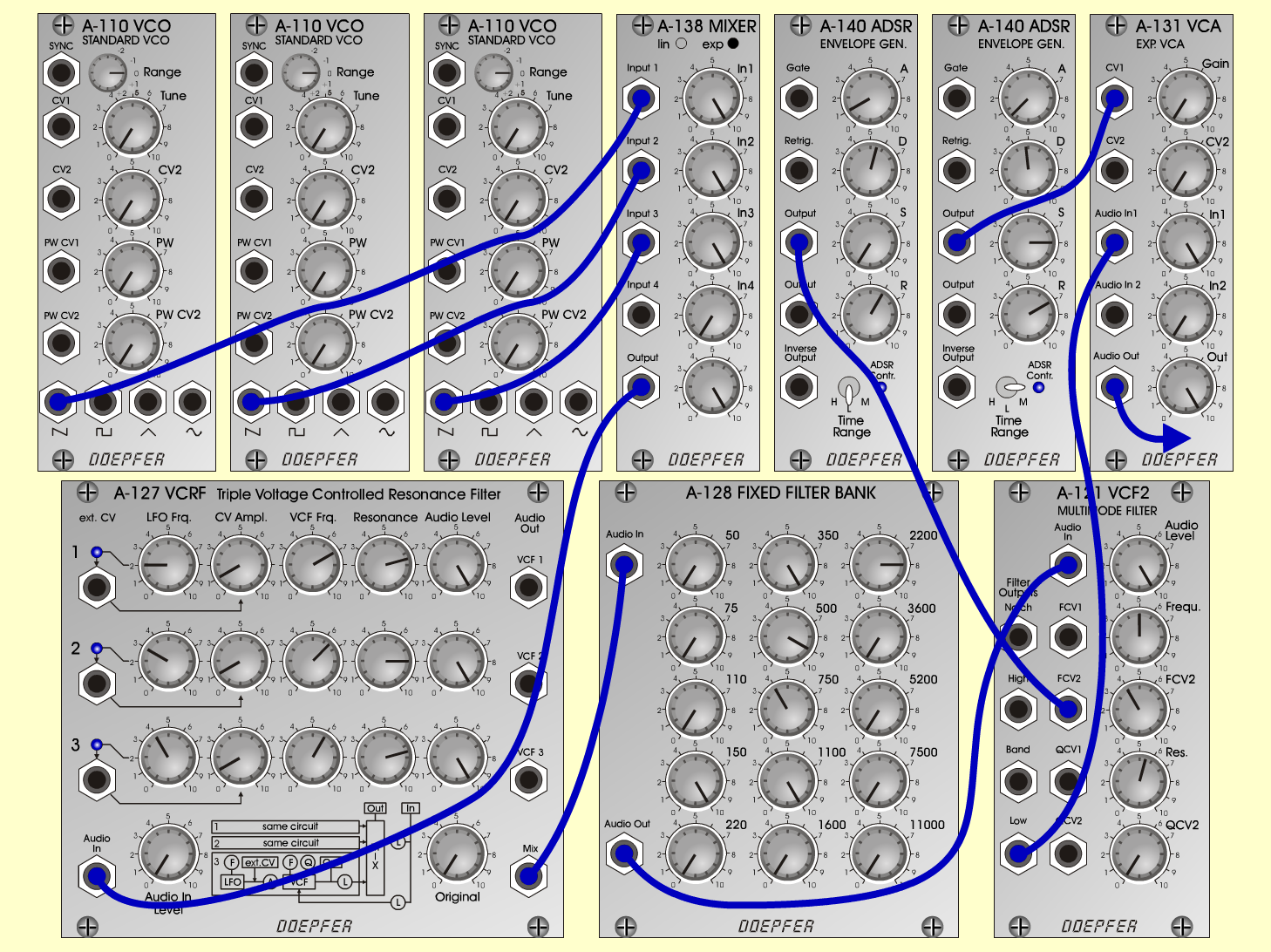Moon-WalkerS y n t hD o e p f e r A - 100 Connections: Settings: A-110/1 (Saw)  <=>  A-138 (Input 1) A-110/2 (Saw)  <=>  A-138 (Input 2) A-110/3 (Saw)  <=>  A-138 (Input 3) A-138 (Output)  <=>  A-127 (Audio In) A-127 (Mix)  <=>  A-128 (Audio In) A-128 (Audio Out)  <=>  A-121 (Audio In) A-121 (Low)  <=>  A-131 (Audio In) Trigger, Gate: KB, Seq <=> A-140 (Gate) A-140/1 (Output)  <=>  A-121 (FCV 2) A-140/2 (Output)  <=>  A-131 (CV 1) Pitch: KB  <=>  A-110/1-3 (CV 1) A-138 (In 1 = 10, In 2 = 10, In 3 = 10, Out = 10) A-127 (Audio In = 8, Original = 0; Row 1: LFO Freq = 2, CV Ampl = 1, VCF Frq = 7, Res = 7.5, Audio Level = 10; Row 2: LFO Freq = 3, CV Ampl = 1, VCF Frq = 6.5, Res = 8, Audio Level = 10; Row 3: LFO Freq = 4, CV Ampl = 1, VCF Frq = 6, Res = 7.5, Audio Level = 10) A-128 (50 = 0, 75 = 0, 110 = 0, 150 = 10, 220 = 0, 350 = 10, 700 = 9, 750 = 4, 1100 = 10, 1600 = 0, 2220 = 8, 3600 = 0, 5220 = 0, 7700 = 0, 11000 = 0) A-121 (Audio Level = 10, Freq = 5, FCV 2 = 4, Res = 5.5) A-140/1 (A = 1, D = 5.5, S = 0, R = 6, Range = L) A-140/2 (A = 0.5, D = 4.8, S = 8, R = 7, Range = M) A-131 (Gain = 0, Audio In 1 = 10,  Audio Out = 10) Notes: Tune: A-110/1 => + 9 cents (e.g. 263.0 Hz) A-110/2 => +/- 0 cents  (e.g. 261.6 Hz) A-110/3 => - 9 cents  (e.g. 260.2 Hz) Josef MuellerSound samples Moonwalker Piece Pachelbel: Canon in D     2./3. instr.: Strings / Harpsichord Moonwalker (65 Hz) Moonwalker (130 Hz)